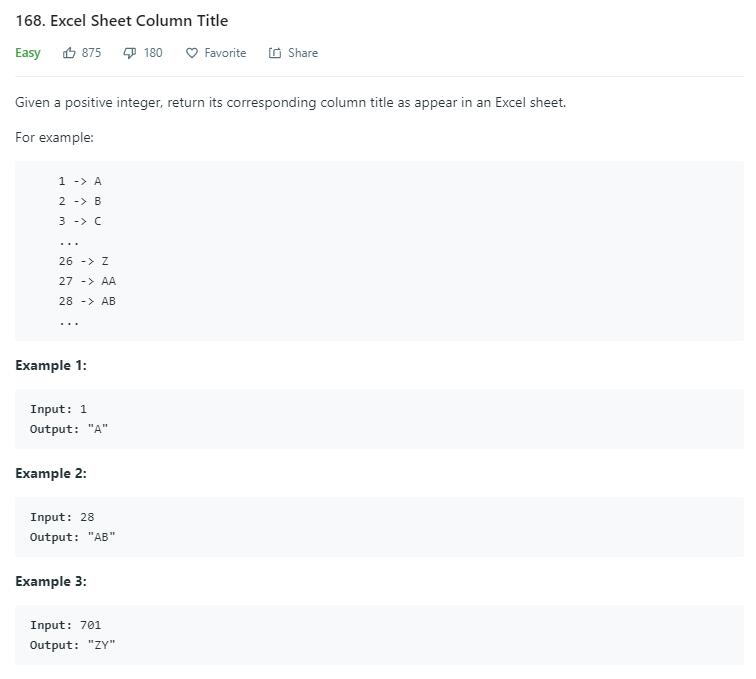# 题目描述（简单难度）# 解法一

16 进制中，09 还是正常的数字，然后增加字母 A 表示 10，字母 B 表示 11... 以此类推，直到 F 表示 15，所以我们各个位的取值范围是 0 - 15

$10\times16^2+1\times16^1+15\times16^0=2591$

$...x_4\times16^3+x_3\times16^2+x_2\times16^1+x_1\times16^0=2591$

$x_1=2591mod16=15$

$...x_4\times16^2+x_3\times16^1+x_2\times16^0=2591/16=161$

public String convertToTitle(int n) {
StringBuilder sb = new StringBuilder();
while (n > 0) {
int c = n % 26;
sb.insert(0, (char) ('A' + c - 1));
n /= 26;
}
return sb.toString();
}


$...x_4\times26^3+x_3\times26^2+x_2\times26^1+x_1\times26^0=2591$

$...x_4\times26^2+x_3\times26^1+x_2\times26^0+1=2591/26$

$...x_4\times26^3+x_3\times26^2+x_2\times26^1+(x_1-1)\times26^0=2591 - 1$

public String convertToTitle(int n) {
StringBuilder sb = new StringBuilder();
while (n > 0) {
int c = n % 26;
if(c == 0){
c = 26;
n -= 1;
}
sb.insert(0, (char) ('A' + c - 1));
n /= 26;
}
return sb.toString();
}